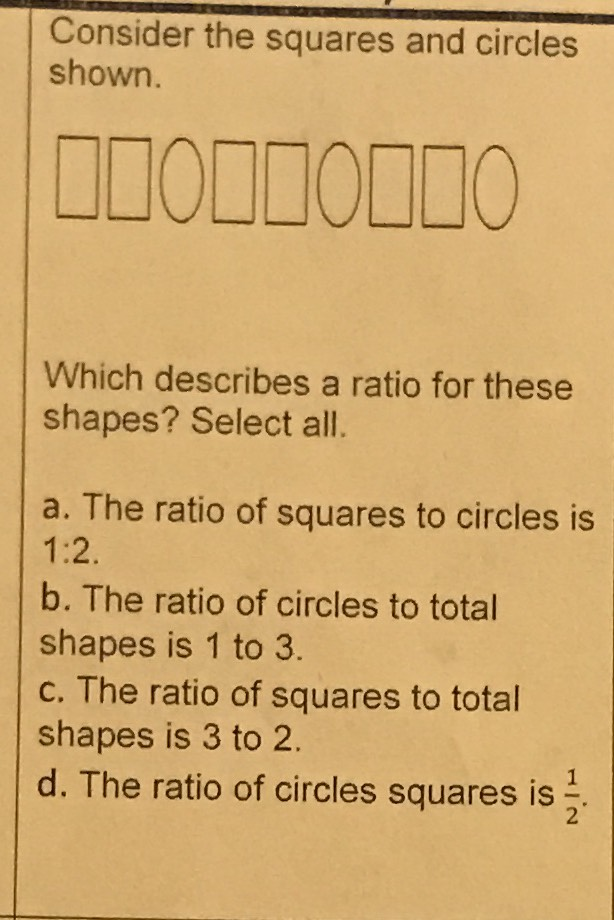### Still have math questions?

Algebra
QuestionConsider the squares and circles shown. Which describes a ratio for these shapes? Select all.

a. The ratio of squares to circles is $$1 : 2$$ .

b. The ratio of circles to total shapes is $$1$$ to $$3$$ .

c. The ratio of squares to total shapes is $$3$$ to $$2$$ .

d. The ratio of circles squares is $$\frac { 1 } { 2 }$$